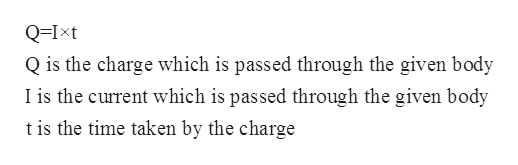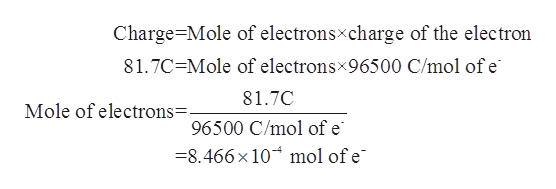# Suppose a current of 0.950A flows through a copper wire for 86. seconds. Calculate how many moles of electrons travel through the wire.Be sure your answer has the correct unit symbol and round your answer to 2 significant digits.

Question
97 views

Suppose a current of 0.950A flows through a copper wire for

86. seconds. Calculate how many moles of electrons travel through the wire.

check_circle

Step 1

The quantity of electric charge that flows through the given solution or electrical wire is equal to the product of the time and current which flows through it.help_outlineImage TranscriptioncloseQ=Ixt Q is the charge which is passed through the given body I is the current which is passed through the given body t is the time taken by the charge fullscreen
Step 2

The current which flows through the copper wire is given to be 0.950 A or 0.950 C/s.

Time taken by the current to flow through the wire is given to be 86 seconds.

The electric charge can be calculated as follows:

Step 3

The number of moles of electrons can ...help_outlineImage TranscriptioncloseCharge Mole of electronsxcharge of the electron 81.7C Mole of electronsx96500 C/mol of e 81.7C Mole of electrons=. 96500 C/mol of e =8.466x 10 mol of e fullscreen

### Want to see the full answer?

See Solution

#### Want to see this answer and more?

Solutions are written by subject experts who are available 24/7. Questions are typically answered within 1 hour.*

See Solution
*Response times may vary by subject and question.
Tagged in

### Physical Chemistry